# Math in Focus Grade 6 Chapter 14 Review Test Answer Key

This handy Math in Focus Grade 6 Workbook Answer Key Chapter 14 Review Test detailed solutions for the textbook questions.

## Math in Focus Grade 6 Course 1 B Chapter 14 Review Test Answer Key

Concepts and Skills

Question 1.
The data set shows nine students’ scores in a science quiz. 9, 6, 6, 5, 9, 10, 1,4, 10
Find the mean and median score.
mean = 6$$\frac{2}{3}$$
median = 6

Explanation:
To find the mean: The sum of the values by adding them all up.
9 + 6 + 6 + 5 + 9 + 10 + 1 + 4 +10 = 60
Divide the sum by the number of values in the data set.
60 ÷ 9
Simplify as = 20 ÷ 3 = 6$$\frac{2}{3}$$
To find the median: Arrange the data points from smallest to largest.
Given set of quiz scores are {9, 6, 6, 5, 9, 10, 1,4, 10}
Ascending order of the scores are {1, 4, 5, 6, 6, 9, 9, 10, 10}
If the number of data points is odd, the median is the middle data point in the list.
So, the median is 6.

Question 2.
The mean of a set of four numbers is 3.5. If a fifth number, x, is added to the data set, the mean becomes 4. Find the value of x.
x = 6

Explanation:
Given that,
The mean of a set of four numbers is 3.5.
Total = 3.5 x 4 = 14
If a fifth number, x, is added to the data set, the mean becomes 4.
(14 + x) ÷ 5 = 4
14 + x = 20
x = 20 – 14
x = 6

Make a dot plot to show the data. Use your dot plot to answer the question.

Question 3.
The data set shows the number of vehicles at a highway intersection during morning rush hour on 15 working days.
12, 11, 4, 6, 9, 11, 4, 6, 12, 16, 11, 10, 8, 4, 5
Find the mean, median, and mode of the data set.
mean = 8.6
median = 9
mode = 4 and 11

Explanation:
To find the mean:
The sum of the values by adding them all up.
12 + 11 + 4 + 6 + 9 + 11 + 4 + 6 + 12 + 16 + 11 + 10 + 8 + 4 + 5 = 129
Divide the sum by the number of values in the data set.
129 ÷ 15
To find the median:
Arrange the data points from smallest to largest.
Given set of quiz scores are {12, 11, 4, 6, 9, 11, 4, 6, 12, 16, 11, 10, 8, 4, 5}
Ascending order of the scores are {4, 4, 4, 5, 6, 6, 8, 9, 10, 11, 11, 11, 12, 12, 16}
If the number of data points is odd, the median is the middle data point in the list.
So, the median is 9.
To find mode:
The mode is simply the number that appears most often within a data set.
So, 4 and 11 appears 3 times in the set.

Problem Solving

The data set shows the amount of money 10 children spent in a week.
$16,$13, $11,$19, $17,$28, $15,$11, $13,$11

Question 4.
Find the mean and median amount of money spent.
mean = 15.4
median = $16 Explanation: Mean: The sum of the values by adding them all up.$16 + $13 +$11 + $19 +$17 + $28 +$15 + $11 +$13 + $11 = 154 Divide the sum by the number of values in the data set. 154 ÷ 10 = 15.4 Median: Arrange the data points from smallest to largest. Given set of quiz scores are {$16, $13,$11, $19,$17, $28,$15, $11,$13, $11} Ascending order of the scores are {$11, $11,$11, $13,$13, $15,$16, $17,$19, $28} If the number of data points is even, the median is the average of middle 2 data point in the list.$13 and $15 are the middle points in the data. 13 + 15 = 28 28 ÷ 2 = 16 So, the median is$16.

Question 5.
Which amount of money would you delete from the list if you want the mean to be closer to the median? Explain your answer.
$28 Explanation: Amounts at the extreme have more effect on the mean than the median. Ascending order of the scores are {$11, $11,$11, $13,$13, $15,$16, $17,$19}

Use the data in the table to answer the question.

Question 6.
Three classes in Grade 7 took a geography test last week. The table shows the mean score of the students in each class.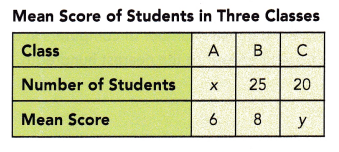The mean score of the students in classes A and B combined is 7.25. The mean score of all the students in the three classes is 6.5. Find the values of x and y.
x = 15
y = 5

Explanation:
The mean score of the students in classes A and B combined is 7.25.
The mean score of all the students in the three classes is 6.5.
The mean = $$\frac{Sum of a set of items}{Number of items}$$
7.25 = $$\frac{6× + 25×8}{25 + x}$$
60x + 200 = 7.25{25 + x}
6x + 200 =7.25x + 181.25
200 – 181.25 = 7.25-6x
18.75 = 1.25x
x = 15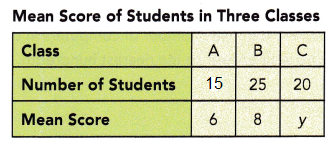Mean
15×6 + 25×8 + 20y = 6.5(15+25+20)
90+200+20y = 6.5 x 60
290 + 20y = 390
20y = 390-290
y = 100/20
y = 5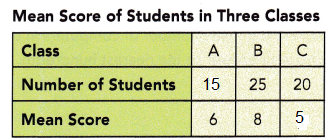Make a dot plot to show the data. Use your dot plot to answer questions 7 and 8.

The table shows the number of goals scored by a soccer team in 15 games.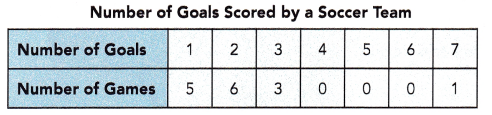Question 7.
Find the mean, median, and mode of the data set.
mean = 2.2
median = 2
mode = 2

Explanation:
The above tabulated data is the table shows the number of goals scored by a soccer team in 15 games.
Mean:
The mean = $$\frac{Sum of a set of items}{Number of items}$$

mean = $$\frac{1×5 + 6×2 + 3×3 + 0×4 + 0×5 + 0×6 + 1×7}{15}$$
mean = $$\frac{5 + 12 + 9 + 7}{15}$$
mean = $$\frac{33}{15}$$
mean = 2.2
Mode
The above observations are:
1, 1, 1, 1, 1, 2, 2,2 2, 2, 2, 3, 3, 3, 7
As 2 has more number the table shows the number of goals scored by a soccer team in 15 games.
Mode is 2
Median:
The above observations are
1, 1, 1, 1, 1, 2, 2,2 2, 2, 2, 3, 3, 3, 7
total 15 observation
median : Middle value is the median of a given data set.
1, 1, 1, 1, 1, 2, 2,2 2, 2, 2, 3, 3, 3, 7
the above data has 15 observations,
2 is in the middle of the above series of numbers.
hence 2 is the median.

Question 8.
Briefly describe the data distribution and relate the measure of center to the distribution.
The mean is to the right of the median. with mean 2.2 as peak.

Explanation:
Measures of central tendency are summary statistics that represent the center point or typical value of a dataset.
The shape of the data distribution is right skewed.
The mean gives more weight to the values on the right than the median does.
So, the mean is to the right of the median. with mean 2.2 as peak.

Use the data in the dot plot to answer questions 9 to 13.

The dot plot shows the results of a survey to find the number of computers in 30 randomly chosen families. Each dot represents 1 family.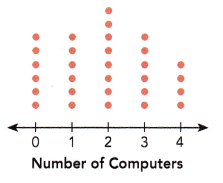Question 9.
What is the modal number of computers?
2

Explanation:
The above dot plot shows the results of a survey of the number of computers in 30 randomly chosen families. Each dot represents 1 family.
8 families with 2 computes
So, the mode of the above data is 2
as 2 computers having families are more.

Question 10.
What is the mean number of computers? Round your answer to the hundredths place.
Mean = 1.9
The nearest hundredth place is 2.

Explanation:
The mean = $$\frac{Sum of a set of items}{Number of items}$$
mean = $$\frac{0×6 + 1×6 +2×8 + 3×6 + 4×4}{30}$$
mean = $$\frac{0 + 6 +16 + 18 + 16 }{30}$$
mean = $$\frac{56}{30}$$
mean = 1.9

Question 11.
What is the median number of computers?
median = 2

Explanation:
The above observations are:
0, 0, 0, 0, 0, 0, 1, 1, 1, 1, 1, 1, 2, 2, 2, 2, 2, 2, 2, 2, 3, 3, 3, 3, 3, 3, 4, 4, 4, 4
total 30 observation
median : Middle value is the median of a given data set.
0, 0, 0, 0, 0, 0, 1, 1, 1, 1, 1, 1, 2, 2, 2, 2, 2, 2, 2, 2, 3, 3, 3, 3, 3, 3, 4, 4, 4, 4
the above data has 30 observations,
So, 2, 2 in the middle of the order sequence is the median.
the average of (2 + 2)/2 = 4/2 = 2
hence 2 is the median.

Question 12.
Briefly describe the data distribution and relate the measure of center to the shape of the dot plot shown.
A measure of center is called a measure of central tendency of the data plot.

Explanation:
Given, The dot plot shows the results of a survey to find the number of computers in 30 randomly chosen families. Each dot represents 1 family.Center describes a typical value of a data point.
Two measures of center are mean and median.
The shape of the data distribution is right skewed.
Hence, the mean and median are 2.

Question 13.
A similar survey is carried out on another 15 randomly chosen families and the mean number of computers is found to be 2. If the two data sets are combined, find the mean number of computers in the combined data set. Round your answer to the nearest hundredth.
mean = 1.86
nearest hundredth = 2

Explanation:
Number of Computers in 1st set
0×6 + 1×6 +2×8 + 3×6 + 4×4 = 56
Number of families in 1st set = 30
Number of Computers in 2nd set = x
Number of families in 2nd set = 15
Mean of 2nd set = 2
mean = $$\frac{Total number of computes in 2nd set}{Total number of families}$$
2 = $$\frac{x}{15}$$
x = 30
Total number of computers = 30 + 56 = 86
Total number of families = 15 +30 = 45

mean = $$\frac{86}{45}$$
mean = 1.91
Round the answer to the nearest hundredth.
mean 1.9